# 【每周FPGA案例】 至简设计系列_简易计算器

1.1 总体设计

1.1.1 概述

1.1.2 设计目标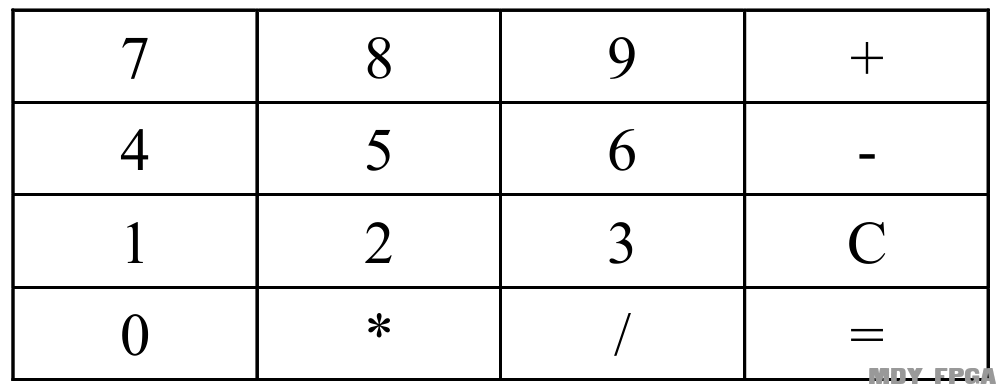1、  计算器通过矩阵键盘模拟按键输入，并通过数码管显示。
2、  计算器有“0、1、2、3、4、5、6、7、8、9、+、-、*、/、C、=”共16个按键。
3、  计算器不支持输入负数，运算结果支持负数但不支持小数。
4、  运算数1、运算数2以及运算结果最大支持8位。其中，运算数1和运算结果的位数包括符号位“-”。
5、  运算数1和运算数2的默认值为0.
6、  计算器支持连续运算，允许在输入运算数2后按下运算符，或者得出运算结果后按下运算符。
7、  当运算结果溢出时，数码管显示8个F。
8、  当操作数1或者操作数2的长度溢出时，蜂鸣器会响。

1.1.3 系统结构框图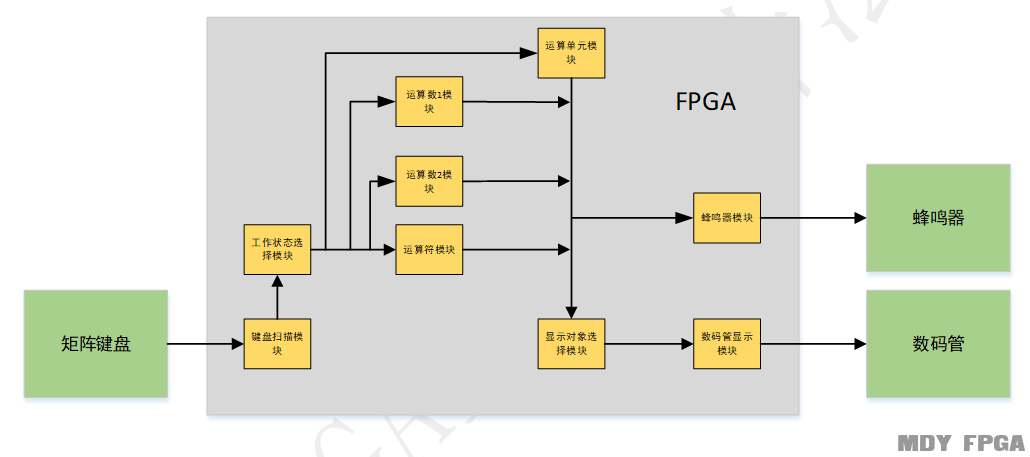1.1.4模块功能

1、将外来异步信号打两拍处理，将异步信号同步化。
2、实现20ms按键消抖功能。
3、实现矩阵键盘的按键检测功能，并输出有效按键信号。

1、  根据接收的不同的按键信号，判断和决定计算器的工作状态。共有5种状态，输入运算数1（OP_1）、运算符（OPER）、输入运算数2（OP_2）、输出结果（RESULT）、结果错误（ERROR）

&#216;  运算数1模块实现功能
1、  当计算器处于运算数1状态下，任何连续输入的数字（不超过8位）都将存放在该模块中，作为运算数1.
2、  当运算数已经到达8位时，此时无论输入任何数字，运算数1不变。
3、  当计算器经过一次运算后（按下等号或者在运算数2状态下按下运算符），运算数去存放结果result。

&#216;  运算符模块实现功能
1、  保存最新按下的运算符。

&#216;  运算数2模块实现功能
1、  当计算器处于运算数2状态下，任何连续输入的数字（不超过8位）都将存放在该模块中，作为运算数2，默认值为0。
2、  当运算数2已经到达8（包括符号位“-”），此时无论输入任何数字，运算数2不变。

&#216;  运算单元模块实现功能
1、  当计算器处于运算数2状态下按下运算符或者在任何状态下按下等号时，该模块根据此时运算数1、运算数2以及运算符的值，进行运算。
2、  若运算结果溢出，或者长度大于8位（包括符号位“-”）或者除数为0时，输出8个F。
3、  最多保留运算结果整数部分的8个有效数字，不保留任何小数。

&#216;  显示对象选则模块实现功能
1、  该模块的作用是根据当前计算器的工作状态来选择数码管的显示内容。

&#216;  数码管显示模块实现功能
1、  该模块的作用是对显示对象选择模块的显示数据输出信号进行数码管显示。

&#216;  蜂鸣器模块实现功能
1、  该模块的作用是对各种错误输入或输出进行响铃警告。

1.1.5顶层信号

 信号名 接口方向 定义 clk 输入 系统时钟，50Mhz rst_n 输入 低电平复位信号 Key_col 输入 4位矩阵键盘列信号，默认高电平，开发板按键为普通按键时，不需要该信号 Key_row 输出 4位矩阵键盘行信号，默认低电平，开发板按键为普通按键时，不需要该信号 Segment 输出 8位数码管段选信号 Seg_sel 输出 8位数码管位选信号 beep 输出 1位蜂鸣器控制信号

1.1.6参考代码
1. module  calc_project(
2.     clk     ,
3.     rst_n   ,
4.     key_col ,
5.     key_row ,
6.     seg_sel ,
7.     segment ,
8.     beep
9.     );

10.     parameter   KEY_WID     =   4   ;
11.     parameter   STATE_WID   =   5   ;
12.     parameter   NUM_WID     =   27  ;
13.     parameter   SEG_NUM     =   8   ;
14.     parameter   SEG_WID     =   8   ;

15.     input                       clk         ;
16.     input                       rst_n       ;
17.     input   [KEY_WID-1:0]       key_col     ;
18.     output  [KEY_WID-1:0]       key_row     ;
19.     output  [SEG_NUM-1:0]       seg_sel     ;
20.     output  [SEG_WID-1:0]       segment     ;
21.     output                      beep        ;

22.     wire    [KEY_WID-1:0]       key_num     ;
23.     wire                        key_vld     ;
24.     wire    [KEY_WID-1:0]       key_num_out ;
25.     wire    [KEY_WID-1:0]       key_vld_out ;
26.     wire    [STATE_WID-1:0]     state_c     ;
27.     wire    [NUM_WID-1:0]       op_1        ;
28.     wire                        op_1_err    ;
29.     wire    [KEY_WID-1:0]       oper        ;
30.     wire    [NUM_WID-1:0]       op_2        ;
31.     wire                        op_2_err    ;
32.     wire    [NUM_WID-1:0]       result      ;
33.     wire                        result_err  ;
34.     wire                        result_neg  ;
35.     wire    [SEG_NUM*4-1:0]     display     ;
36.     wire                        display_vld ;

37.     key_scan   key_scan_prj(
38.                             .clk        (clk    )   ,
39.                             .rst_n      (rst_n  )   ,
40.                             .key_col    (key_col)   ,
41.                             .key_row    (key_row)   ,
42.                             .key_out    (key_num)   ,
43.                             .key_vld    (key_vld)
44.                             );

45.     work_state  work_state_prj(
46.                                 .clk        (clk        )   ,
47.                                 .rst_n      (rst_n      )   ,
48.                                 .key_num    (key_num    )   ,
49.                                 .key_vld    (key_vld    )   ,
50.                                 .result_err (result_err )   ,
51.                                 .key_num_out(key_num_out)   ,
52.                                 .key_vld_out(key_vld_out)   ,
53.                                 .state_c    (state_c    )
54.                                 );

55.     op_1    op_1_prj(
56.                         .clk        (clk        )   ,
57.                         .rst_n      (rst_n      )   ,
58.                         .key_num    (key_num_out)   ,
59.                         .key_vld    (key_vld_out)   ,
60.                         .state_c    (state_c    )   ,
61.                         .result     (result     )   ,
62.                         .op_1       (op_1       )   ,
63.                         .op_1_err   (op_1_err   )
64.                     );

65.     oper    oper_prj(
66.                         .clk        (clk        )   ,
67.                         .rst_n      (rst_n      )   ,
68.                         .key_num    (key_num_out)   ,
69.                         .key_vld    (key_vld_out)   ,
70.                         .state_c    (state_c    )   ,
71.                         .oper       (oper       )
72.                     );
73.
74.     op_2    op_2_prj(
75.                         .clk        (clk        )   ,
76.                         .rst_n      (rst_n      )   ,
77.                         .key_num    (key_num_out)   ,
78.                         .key_vld    (key_vld_out)   ,
79.                         .state_c    (state_c    )   ,
80.                         .op_2       (op_2       )   ,
81.                         .op_2_err   (op_2_err   )
82.                     );

83.     result  result_prj(
84.                         .clk        (clk        )   ,
85.                         .rst_n      (rst_n      )   ,
86.                         .key_num    (key_num_out)   ,
87.                         .key_vld    (key_vld_out)   ,
88.                         .state_c    (state_c    )   ,
89.                         .op_1       (op_1       )   ,
90.                         .oper       (oper       )   ,
91.                         .op_2       (op_2       )   ,
92.                         .result     (result     )   ,
93.                         .result_err (result_err )   ,
94.                         .result_neg (result_neg )
95.                     );

96.     display_sel  display_sel_prj(
97.                                 .clk        (clk        )   ,
98.                                 .rst_n      (rst_n      )   ,
99.                                 .state_c    (state_c    )   ,
100.                                 .op_1       (op_1       )   ,
101.                                 .op_2       (op_2       )   ,
102.                                 .result_neg (result_neg )   ,
103.                                 .display    (display    )   ,
104.                                 .display_vld(display_vld)
105.                                 );

106.     segment  segment_prj(
107.                             .rst_n      (rst_n      )   ,
108.                             .clk        (clk        )   ,
109.                             .display    (display    )   ,
110.                             .display_vld(display_vld)   ,
111.                             .seg_sel    (seg_sel    )   ,
112.                             .segment    (segment    )
113.                         );

114.     beep    beep_prj(
115.                         .clk        (clk        )   ,
116.                         .rst_n      (rst_n      )   ,
117.                         .op_1_err   (op_1_err   )   ,
118.                         .op_2_err   (op_2_err   )   ,
119.                         .result_err (result_err )   ,
120.                         .beep       (beep       )
121.                     );

122. endmodule

1.2 键盘扫描模块设计1.2.1接口信号
 信号 接口方向 定义 clk 输入 系统时钟 rst_n 输入 低电平复位信号 key_col 输入 矩阵键盘列输入信号 Key_row 输出 矩阵键盘行输出信号 Key_out 输出 按键位置输出信号，key_vld有效时，该信号有效。 Key_vld 输出 按键有效指示信号，高电平有效

1.2.2 设计思路

1.2.3参考代码
1. always  @(posedge clk or negedge rst_n)begin
2.     if(rst_n==1'b0)begin
3.         key_col_ff0 <= 4'b1111;
4.         key_col_ff1 <= 4'b1111;
5.     end
6.     else begin
7.         key_col_ff0 <= key_col    ;
8.         key_col_ff1 <= key_col_ff0;
9.     end
10. end

11. always @(posedge clk or negedge rst_n) begin
12.     if (rst_n==0) begin
13.         shake_cnt <= 0;
14.     end
16.         if(end_shake_cnt)
17.             shake_cnt <= 0;
18.         else
19.             shake_cnt <= shake_cnt+1 ;
20.    end
21. end
23. assign end_shake_cnt = add_shake_cnt  && shake_cnt == TIME_20MS-1 ;

24. always  @(posedge clk or negedge rst_n)begin
25.     if(rst_n==1'b0)begin
26.         state_c <= CHK_COL;
27.     end
28.     else begin
29.         state_c <= state_n;
30.     end
31. end

32. always  @(*)begin
33.     case(state_c)
34.         CHK_COL: begin
35.                      if(col2row_start )begin
36.                          state_n = CHK_ROW;
37.                      end
38.                      else begin
39.                          state_n = CHK_COL;
40.                      end
41.                  end
42.         CHK_ROW: begin
43.                      if(row2del_start)begin
44.                          state_n = DELAY;
45.                      end
46.                      else begin
47.                          state_n = CHK_ROW;
48.                      end
49.                  end
50.         DELAY :  begin
51.                      if(del2wait_start)begin
52.                          state_n = WAIT_END;
53.                      end
54.                      else begin
55.                          state_n = DELAY;
56.                      end
57.                  end
58.         WAIT_END: begin
59.                      if(wait2col_start)begin
60.                          state_n = CHK_COL;
61.                      end
62.                      else begin
63.                          state_n = WAIT_END;
64.                      end
65.                   end
66.        default: state_n = CHK_COL;
67.     endcase
68. end
69. assign col2row_start = state_c==CHK_COL  && end_shake_cnt;
70. assign row2del_start = state_c==CHK_ROW  && row_index==3 && end_row_cnt;
71. assign del2wait_start= state_c==DELAY    && end_row_cnt;
72. assign wait2col_start= state_c==WAIT_END && key_col_ff1==4'hf;

73. always  @(posedge clk or negedge rst_n)begin
74.     if(rst_n==1'b0)begin
75.         key_row <= 4'b0;
76.     end
77.     else if(state_c==CHK_ROW)begin
78.         key_row <= ~(1'b1 << row_index);
79.     end
80.     else begin
81.         key_row <= 4'b0;
82.     end
83. end

84. always @(posedge clk or negedge rst_n) begin
85.     if (rst_n==0) begin
86.         row_index <= 0;
87.     end
89.         if(end_row_index)
90.             row_index <= 0;
91.         else
92.             row_index <= row_index+1 ;
93.    end
94.    else if(state_c!=CHK_ROW)begin
95.        row_index <= 0;
96.    end
97. end
98. assign add_row_index = state_c==CHK_ROW && end_row_cnt;
99. assign end_row_index = add_row_index  && row_index == 4-1 ;

100. always @(posedge clk or negedge rst_n) begin
101.     if (rst_n==0) begin
102.         row_cnt <= 0;
103.     end
105.         if(end_row_cnt)
106.             row_cnt <= 0;
107.         else
108.             row_cnt <= row_cnt+1 ;
109.    end
110. end
111. assign add_row_cnt = state_c==CHK_ROW || state_c==DELAY;
112. assign end_row_cnt = add_row_cnt  && row_cnt == 16-1 ;

113. always  @(posedge clk or negedge rst_n)begin
114.     if(rst_n==1'b0)begin
115.         key_col_get <= 0;
116.     end
117.     else if(state_c==CHK_COL && end_shake_cnt ) begin
118.         if(key_col_ff1==4'b1110)
119.             key_col_get <= 0;
120.         else if(key_col_ff1==4'b1101)
121.             key_col_get <= 1;
122.         else if(key_col_ff1==4'b1011)
123.             key_col_get <= 2;
124.         else
125.             key_col_get <= 3;
126.     end
127. end

128. always  @(posedge clk or negedge rst_n)begin
129.     if(rst_n==1'b0)begin
130.         key_out <= 0;
131.     end
132.     else if(state_c==CHK_ROW && end_row_cnt)begin
133.         key_out <= {row_index,key_col_get};
134.     end
135.     else begin
136.         key_out <= 0;
137.     end
138. end

139. always  @(posedge clk or negedge rst_n)begin
140.     if(rst_n==1'b0)begin
141.         key_vld <= 1'b0;
142.     end
143.     else if(state_c==CHK_ROW && end_row_cnt && key_col_ff1[key_col_get]==1'b0)begin
144.         key_vld <= 1'b1;
145.     end
146.     else begin
147.         key_vld <= 1'b0;
148.     end
149. end

1.3 工作状态选择模块设计1.3.1接口信号
 信号 接口方向 定义 clk 输入 系统时钟 rst_n 输入 低电平复位信号 key_vld 输入 按键按下指示信号 Key_num 输入 按键位置输入信号，key_vld有效时，该信号有效 Result_err 输入 运算结果错误指示信号 Key_num_out 输出 计算器按下位置输出信号，key_vld_out有效时，该信号有效。 Key_vld_out 输出 计算器按键按下有效指示信号，高电平有效。 State_c 输出 计算器工作状态指示信号

1.3.2设计思路OP_1状态下：
A．  按下等号，跳到RESULT状态；
B．  按下运算符，跳到OPER状态；
OPER状态下：
A．  按下数字，跳到OP_2状态；
B．  按下等号，跳到RESULT状态；
OP_2状态下：
A．  按下等号，跳到RESULT状态；
B．  按下运算符，跳到OPER状态；
RESULT状态下：
A．  按下数字，跳到OP_1状态；
B．  按下运算符，跳到OPER状态；
C．  按下等号，停留在RESULT状态；
ERROR状态下：
A．  按下数字，跳到OP_1状态；
B．  按下其他按键，停留在ERROR状态；

1.3.3参考代码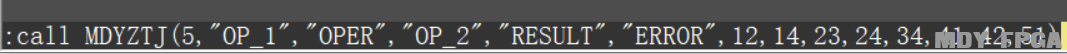1. always  @(*)begin
2.     case(key_num)
3.         4'd0   :key_num_chg = 4'd7   ;
4.         4'd1   :key_num_chg = 4'd8   ;
5.         4'd2   :key_num_chg = 4'd9   ;
6.         4'd3   :key_num_chg = 4'd10  ;
7.         4'd7   :key_num_chg = 4'd11  ;
8.         4'd8   :key_num_chg = 4'd1   ;
9.         4'd9   :key_num_chg = 4'd2   ;
10.         4'd10  :key_num_chg = 4'd3   ;
11.         4'd11  :key_num_chg = 4'd14  ;
12.         4'd12  :key_num_chg = 4'd0   ;
13.         4'd13  :key_num_chg = 4'd12  ;
14.         4'd14  :key_num_chg = 4'd13  ;
15.         default:key_num_chg = key_num;
16.     endcase
17. end

18. assign  key_num_en = (key_num_chg==0 || key_num_chg==1 || key_num_chg==2 || key_num_chg==3 || key_num_chg==4 || key_num_chg==5 || key_num_chg==6 || key_num_chg==7 || key_num_chg==8 || key_num_chg==9) && key_vld==1;
19. assign  key_op_en = (key_num_chg==10 || key_num_chg==11 || key_num_chg==12 || key_num_chg==13) && key_vld==1;
20. assign  key_cal_en = key_num_chg==15 && key_vld==1;
21. assign  key_back_en = key_num_chg==14 && key_vld==1;

22. always @(posedge clk or negedge rst_n) begin
23.     if (rst_n==0) begin
24.         state_c <= OP_1 ;
25.     end
26.     else begin
27.         state_c <= state_n;
28.    end
29. end

30. always @(*) begin
31.     if(result_err)begin
32.        state_n = ERROR;
33.    end
34.    else begin
35.        case(state_c)
36.         OP_1 :begin
37.             if(op_12oper_start)
38.                 state_n = OPER ;
39.             else if(op_12result_start)
40.                 state_n = RESULT ;
41.             else
42.                 state_n = state_c ;
43.         end
44.         OPER :begin
45.             if(oper2op_2_start)
46.                 state_n = OP_2 ;
47.             else if(oper2result_start)
48.                 state_n = RESULT ;
49.             else
50.                 state_n = state_c ;
51.         end
52.         OP_2 :begin
53.             if(op_22oper_start)
54.                 state_n = OPER ;
55.             else if(op_22result_start)
56.                 state_n = RESULT ;
57.             else
58.                 state_n = state_c ;
59.         end
60.         RESULT :begin
61.             if(result2op_1_start)
62.                 state_n = OP_1 ;
63.             else if(result2oper_start)
64.                 state_n = OPER ;
65.             else
66.                 state_n = state_c ;
67.         end
68.         ERROR :begin
69.             if(error2op_1_start)
70.                 state_n = OP_1 ;
71.             else
72.                 state_n = state_c ;
73.         end
74.         default : state_n = OP_1 ;
75.     endcase
76. end
77. end

78. assign op_12oper_start   = state_c==OP_1   && key_op_en ;
79. assign op_12result_start = state_c==OP_1   && key_cal_en;
80. assign oper2op_2_start   = state_c==OPER   && key_num_en;
81. assign oper2result_start = state_c==OPER   && key_cal_en;
82. assign op_22oper_start   = state_c==OP_2   && key_op_en ;
83. assign op_22result_start = state_c==OP_2   && key_cal_en;
84. assign result2op_1_start = state_c==RESULT && key_num_en;
85. assign result2oper_start = state_c==RESULT && key_op_en ;
86. assign error2op_1_start  = state_c==ERROR  && key_num_en;

87. always  @(posedge clk or negedge rst_n)begin
88.     if(rst_n==1'b0)begin
89.         key_num_out <= 0;
90.     end
91.     else begin
92.         key_num_out <= key_num_chg;
93.     end
94. end

95. always  @(posedge clk or negedge rst_n)begin
96.     if(rst_n==1'b0)begin
97.         key_vld_out <= 0;
98.     end
99.     else begin
100.         key_vld_out <= key_vld;
101.     end
102. end

1.4 运算数1模块设计1.4.1接口信号
 信号 接口方向 定义 clk 输入 系统时钟 rst_n 输入 低电平复位信号 Key_num_out 输入 计算器按下位置输出信号，key_vld_out有效时，该信号有效。 Key_vld_out 输入 计算器按键按下有效指示信号，高电平有效。 State_c 输入 计算器工作状态指示信号 Op_1 输出 运算数1输出信号 Result 输入 运算结果输出信号 Op_1_err 输出 运算数1溢出信号

1.4.2设计思路

1、 该信号为高时，表示完成一次计算过程得到了结果。初始状态为低电平；
2、 当输入操作数2后又按下了等号或者其他操作符的时候，变为高电平，所以变高的条件为(state_c_ff==OP_2 && state_c==OPER) || state_c==RESULT；
3、 当处在操作数1状态时，为低电平，所以变低的条件为state_c==OP_1。其他情况保持不变。

1、 该信号表示运算数1要输出的值。初始状态为0；
2、 在结果错误状态的时候，给一个不超过范围的任意值，此代码中给的10；
3、 在得到计算结果或者计算结果错误的时候，输入数字，输出为按下按键的对应值（key_num_out）；
4、 在输入操作数1之后，按下退格键，op_1输出的值除以10进行取整；
5、 在输入操作数1状态下通过键盘输入数字，需要判断是否超过显示范围，如果没有超过的话就需要将当前op_1的值乘以10，然后加上按下的数字的值，进行输出；
6、 当计算完成时，即flag_calc==1，操作数1输出计算的结果result；
7、 其他时侯操作数1保持不变。

1、 初始状态为0，表示没有溢出。
2、 当一直处于操作数1状态，按下键盘输入数字之后，操作数1的值溢出了，则将运算数1溢出信号拉高。
3、 其他时刻保持为低电平。
1. assign  key_num_en = (key_num==0 || key_num==1 || key_num==2 || key_num==3 || key_num==4 || key_num==5 || key_num==6 || key_num==7 || key_num==8 || key_num==9) && key_vld==1;
2. assign  key_op_en = (key_num==10 || key_num==11 || key_num==12 || key_num==13) && key_vld==1;
3. assign  key_cal_en = key_num==15 && key_vld==1;
4. assign  key_back_en = key_num==14 && key_vld==1;

5. always  @(posedge clk or negedge rst_n)begin
6.     if(rst_n==1'b0)begin
7.         state_c_ff <= 0;
8.     end
9.     else begin
10.         state_c_ff <= state_c;
11.     end
12. end

13. always  @(posedge clk or negedge rst_n)begin
14.     if(rst_n==1'b0)begin
15.         op_2 <= 0;
16.     end
17.     else if(state_c==OPER)begin
18.         op_2 <= 0;
19.     end
20.     else if(state_c_ff==OPER && state_c==OP_2)begin
21.         op_2 <= key_num;
22.     end
23.     else if(state_c==OP_2 && key_back_en==1)begin
24.         op_2 <= op_2 / 10;
25.     end
26.     else if(state_c==OP_2 && key_num_en==1)begin
27.         op_2 <= (op_2>9999999) ? op_2 : (op_2*10+key_num);
28.     end
29.     else begin
30.         op_2 <= op_2;
31.     end
32. end

33. always  @(posedge clk or negedge rst_n)begin
34.     if(rst_n==1'b0)begin
35.         op_2_err <= 0;
36.     end
37.     else if(state_c==OP_2 && key_num_en==1 && op_2>9999999)begin
38.         op_2_err <= 1'b1;
39.     end
40.     else begin
41.         op_2_err <= 1'b0;
42.     end
43. end

44. endmodule

1.4.3参考代码

1.5 运算符模块设计1.5.1接口信号
 信号 接口方向 定义 clk 输入 系统时钟 rst_n 输入 低电平复位信号 Key_num_out 输入 计算器按下位置输出信号，key_vld_out有效时，该信号有效。 Key_vld_out 输入 计算器按键按下有效指示信号，高电平有效。 State_c 输入 计算器工作状态指示信号 oper 输出 运算符输出信号

1.5.2设计思路

1、 当“加”“减”“乘”“除”四个按键的任意一个被按下之后，该信号置为高电平；
2、 当“加”“减”“乘”“除”四个按键没有一个被按下的时候，该信号置为低电平。

1、 当处于操作数1状态时，输出0；
2、 当“加”“减”“乘”“除”任意按键被按下之后，输出该按键对应的值；
3、 其他时候保持不变；

1.5.3参考代码
1. assign  key_op_en = (key_num==10 || key_num==11 || key_num==12 || key_num==13) && key_vld==1;

2. always  @(posedge clk or negedge rst_n)begin
3.     if(rst_n==1'b0)begin
4.         oper <= 0;
5.     end
6.     else if(state_c==OP_1)begin
7.         oper <= 0;
8.     end
9.     else if(key_op_en==1)begin
10.         oper <= key_num;
11.     end
12.     else begin
13.         oper <= oper;
14.     end
15. end

1.6 运算数2模块设计1.6.1接口信号
 信号 接口方向 定义 clk 输入 系统时钟 rst_n 输入 低电平复位信号 Key_num_out 输入 计算器按下位置输出信号，key_vld_out有效时，该信号有效。 Key_vld_out 输入 计算器按键按下有效指示信号，高电平有效。 State_c 输入 计算器工作状态指示信号 Op_2 输出 运算数2输出信号 Op_2_err 输出 运算数2溢出信号

1.6.2设计思路

1、 该信号表示运算数2要输出的值。初始状态为0；
2、 在运算符状态下，此时数码管不显示运算数2的值，让它输出0；
3、 输入运算符之后，之后再输入的就是运算数2的值，此时运算数2就等于按下按键所对应的数值。
4、 在输入运算数2之后，按下退格键，运算数2的值除以10进行取整；
5、 在输入运算数2状态下通过键盘输入数字，需要判断是否超过显示范围，如果没有超过的话就需要将当前运算数2的值乘以10，然后加上按下的数字的值，进行输出；
6、 其他时侯运算数2保持不变。

1、 初始状态为0，表示没有溢出。
2、 当一直处于运算数2状态，按下键盘输入数字之后，运算数2的值溢出了，则将运算数2溢出信号拉高。
3、 其他时刻保持为低电平。

1.6.3参考代码
1. assign  key_num_en = (key_num==0 || key_num==1 || key_num==2 || key_num==3 || key_num==4 || key_num==5 || key_num==6 || key_num==7 || key_num==8 || key_num==9) && key_vld==1;
2. assign  key_op_en = (key_num==10 || key_num==11 || key_num==12 || key_num==13) && key_vld==1;
3. assign  key_cal_en = key_num==15 && key_vld==1;
4. assign  key_back_en = key_num==14 && key_vld==1;

5. always  @(posedge clk or negedge rst_n)begin
6.     if(rst_n==1'b0)begin
7.         state_c_ff <= 0;
8.     end
9.     else begin
10.         state_c_ff <= state_c;
11.     end
12. end

13. always  @(posedge clk or negedge rst_n)begin
14.     if(rst_n==1'b0)begin
15.         flag_calc <= 0;
16.     end
17.     else if(state_c==OP_1)begin
18.         flag_calc <= 1'b0;
19.     end
20.     else if(state_c_ff==OP_2 && state_c==OPER || state_c==RESULT)begin
21.         flag_calc <= 1'b1;
22.     end
23.     else begin
24.         flag_calc <= flag_calc;
25.     end
26. end

27. always  @(posedge clk or negedge rst_n)begin
28.     if(rst_n==1'b0)begin
29.         op_1 <= 0;
30.     end
31.     else if(state_c==ERROR)begin
32.         op_1 <= 10;
33.     end
34.     else if((state_c_ff==RESULT || state_c_ff==ERROR) && state_c==OP_1)begin
35.         op_1 <= key_num;
36.     end
37.     else if(state_c==OP_1 && key_back_en==1)begin
38.         op_1 <= op_1 / 10;
39.     end
40.     else if(state_c==OP_1 && key_num_en==1)begin
41.         op_1 <= (op_1>9999999) ? op_1 : (op_1*10+key_num);
42.     end
43.     else if(flag_calc==1)begin
44.         op_1 <= result;
45.     end
46.     else begin
47.         op_1 <= op_1;
48.     end
49. end

50. always  @(posedge clk or negedge rst_n)begin
51.     if(rst_n==1'b0)begin
52.         op_1_err <= 0;
53.     end
54.     else if(state_c==OP_1  && key_num_en==1 && op_1>9999999)begin
55.         op_1_err <= 1'b1;
56.     end
57.     else begin
58.         op_1_err <= 1'b0;
59.     end
60. end

1.7 运算单元模块设计1.7.1接口信号
 信号 接口方向 定义 clk 输入 系统时钟 rst_n 输入 低电平复位信号 Key_num_out 输入 计算器按下位置输出信号，key_vld_out有效时，该信号有效。 Key_vld_out 输入 计算器按键按下有效指示信号，高电平有效。 State_c 输入 计算器工作状态指示信号 oper 输出 运算符输出信号 Op_1 输入 运算数1输入信号 Op_2 输入 运算数2输入信号 Result 输出 运算结果输出信号 Result_err 输出 运算结果错误信号，运算结果溢出或者除数为0时，该信号输出一个时钟周期的高电平 Result_neg 输出 运算结果符号位指示信号，当运算结果为负数时，该信号为高电平

1.7.2设计思路

1、 只有当运算结果为负数的时候，才显示“负号”，因此初始状态为低电平；
2、 当算式输入完成按下等号之后，如果运算符是“减”，并且运算数1小于运算数2，则运算结果为负数，将result_neg信号拉高。
3、 由于该计算器支持连续输入，如果当前计算的结果为负数，接着输入的运算符为“加”，下一次进行加法运算，并且运算数1（此时比较不考虑符号位）小于或等于运算数2，则表示运算结果为正数，此时将result_neg信号拉低。
4、 在进行连续计算的时候，如果得到的结果超过显示上限，要进入错误状态，这个时候符号位指示信号应该为低电平。
5、 无论在计算中得到的结果是正还是负，如果下次输入的为运算数1，都需要result_neg信号为低电平。
6、 由于除法不支持小数显示，只取整数部分，所以当运算结果为负数，并进行除法运算的时候，如果得到的结果为0，不应该显示为“负0”，应当将符号位指示信号置为低电平。

1、 初始状态没有经过计算，自然输出为0。
2、 在进行加法的时候，由于存在连续计算的情况，需要考虑符号位。当符号位指示信号为0，直接将运算数1和运算数2相加即可；当符号位指示信号为1，则需要判断运算数1和运算数2的大小，确保是大的减去小的。
3、 在进行减法的时候，同样需要考虑符号位。当符号位指示信号为0的时候，需要判断运算数1和运算数2的大小，保证大的减去小的；当符号位指示信号位1的时候，直接将运算数1和运算数2相加即可。
4、 乘法运算直接将运算数1和运算数2相乘即可。
5、 在进行除法运算时，由于无法表示小数，因此这里需要采用运算数1除以运算数2取整的方法，即op_1/op_2。

1、  初始状态下，该信号为0，表示没有错误。
2、  得到运算结果后，若继续输入数字，则会进入到运算数1状态，这个时候不进行错误提示。
3、  在运算数2状态下输入运算符，或者在结果不是错误的状态下输出“等号”（表示进行连续计算）。根据输入的运算符进行相应的判断：

1.7.3参考代码
1. assign  key_op_en = (key_num==10 || key_num==11 || key_num==12 || key_num==13) && key_vld==1;
2. assign  key_cal_en = key_num==15 && key_vld==1;
3. assign  calculate = (state_c_ff==OP_2 && state_c==OPER || key_cal_en==1);

4. always  @(posedge clk or negedge rst_n)begin
5.     if(rst_n==1'b0)begin
6.         state_c_ff <= 0;
7.     end
8.     else begin
9.         state_c_ff <= state_c;
10.     end
11. end

12. always  @(posedge clk or negedge rst_n)begin
13.     if(rst_n==1'b0)begin
14.         result <= 0;
15.     end
16.     else if(calculate==1)begin
17.         case(oper)
19.                 if(result_neg==0)
20.                     result <= op_1 + op_2;
21.                 else
22.                     result <= (op_1>op_2) ? (op_1 - op_2) : (op_2 - op_1);
23.             end
24.             DEV:begin
25.                 if(result_neg==0)
26.                     result <= (op_1>op_2) ? (op_1 - op_2) : (op_2 - op_1);
27.                 else
28.                     result <= op_1 + op_2;
29.             end
30.             MUL:begin
31.                 result <= op_1 * op_2;
32.             end
33.             DIV:begin
34.                 result <= op_1 / op_2;
35.             end
36.             default:result <= op_1;
37.         endcase
38.     end
39.     else begin
40.         result <= result;
41.     end
42. end

43. always  @(posedge clk or negedge rst_n)begin
44.     if(rst_n==1'b0)begin
45.         result_neg <= 0;
46.     end
47.     else if(state_c==OP_1)begin
48.         result_neg <= 1'b0;
49.     end
50.     else if(state_c==ERROR)begin
51.         result_neg <= 1'b0;
52.     end
53.     else if(calculate==1 && oper==DEV && op_1<op_2)begin
54.         result_neg <= 1'b1;
55.     end
56.     else if(calculate==1 && result_neg==1 && oper==ADD && op_1<=op_2)begin
57.         result_neg <= 1'b0;
58.     end
59.     else if(result==0)begin
60.         result_neg <= 1'b0;
61.     end
62.     else begin
63.         result_neg <= result_neg;
64.     end
65. end

66. always  @(posedge clk or negedge rst_n)begin
67.     if(rst_n==1'b0)begin
68.         result_err <= 0;
69.     end
70.     else if(state_c==OP_1)begin
71.         result_err <= 1'b0;
72.     end
73.     else if((state_c_ff==OP_2 && state_c==OPER) || (key_cal_en==1 && state_c_ff!=ERROR))begin
74.         case(oper)
76.                 if(result_neg==0)
77.                     result_err <= (op_1+op_2)>9999_9999 ? 1'b1 : 1'b0;
78.                 else
79.                     result_err <= 1'b0;
80.             end
81.             DEV:begin
82.                 if(result_neg==1)
83.                     result_err <= (op_1+op_2)>999_9999 ? 1'b1 : 1'b0;
84.                 else if(op_2>op_1)
85.                     result_err <= (op_2-op_1)>999_9999 ? 1'b1 : 1'b0;
86.                 else
87.                     result_err <= 1'b0;
88.             end
89.             MUL:begin
90.                 if(result_neg==1)
91.                     result_err <= (op_1*op_2)>999_9999 ? 1'b1 : 1'b0;
92.                 else
93.                     result_err <= (op_1*op_2)>9999_9999 ? 1'b1 : 1'b0;
94.             end
95.             DIV:begin
96.                 if(op_2==0)
97.                     result_err <= 1'b1;
98.                 else
99.                     result_err <= 1'b0;
100.             end
101.             default:result_err <= 1'b0;
102.         endcase
103.     end
104.     else begin
105.         result_err <= 1'b0;
106.     end
107. end

1.8 显示对象选择模块设计1.8.1接口信号
 信号 接口方向 定义 clk 输入 系统时钟 rst_n 输入 低电平复位信号 Op_1 输入 运算数1输入信号 Op_2 输入 运算数2输入信号 State_c 输入 计算器工作状态指示信号 Result_neg 输入 运算结果符号位指示信号 Disply 输出 显示数据输出信号 Display_vld 输出 显示数据有效指示信号

1.8.2设计思路

1、  复位后，该模块输出0；
2、  当计算器处于OP_1状态下，该模块选择输出运算数1。
3、  当计算器处于OPER状态下，该模块选择输出运算数1。
4、  当计算器处于OP_2状态下，该模块选择输出运算数2。
5、  当计算器处于RESULT状态下，该模块选择输出运算数1。
6、  当计算器处于ERROR状态下，该模块选择输出8个F。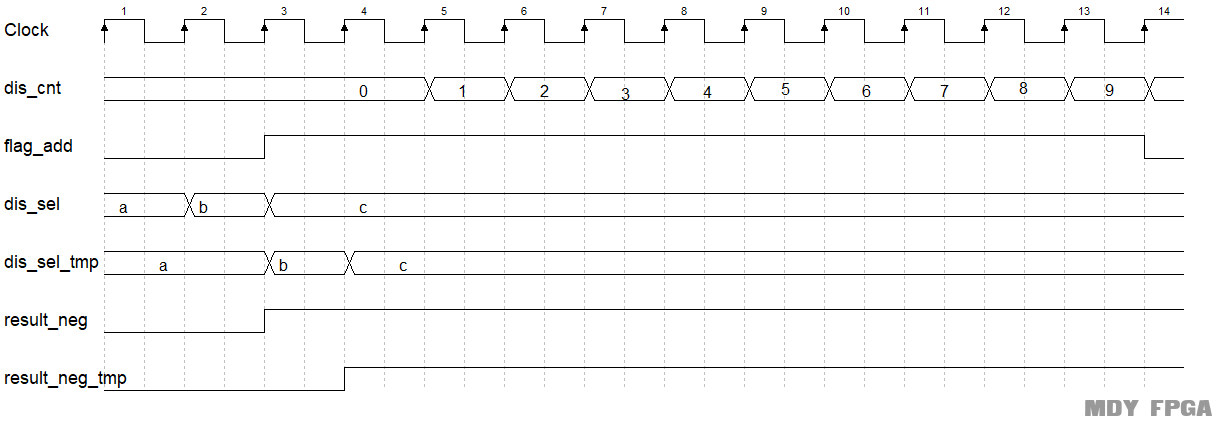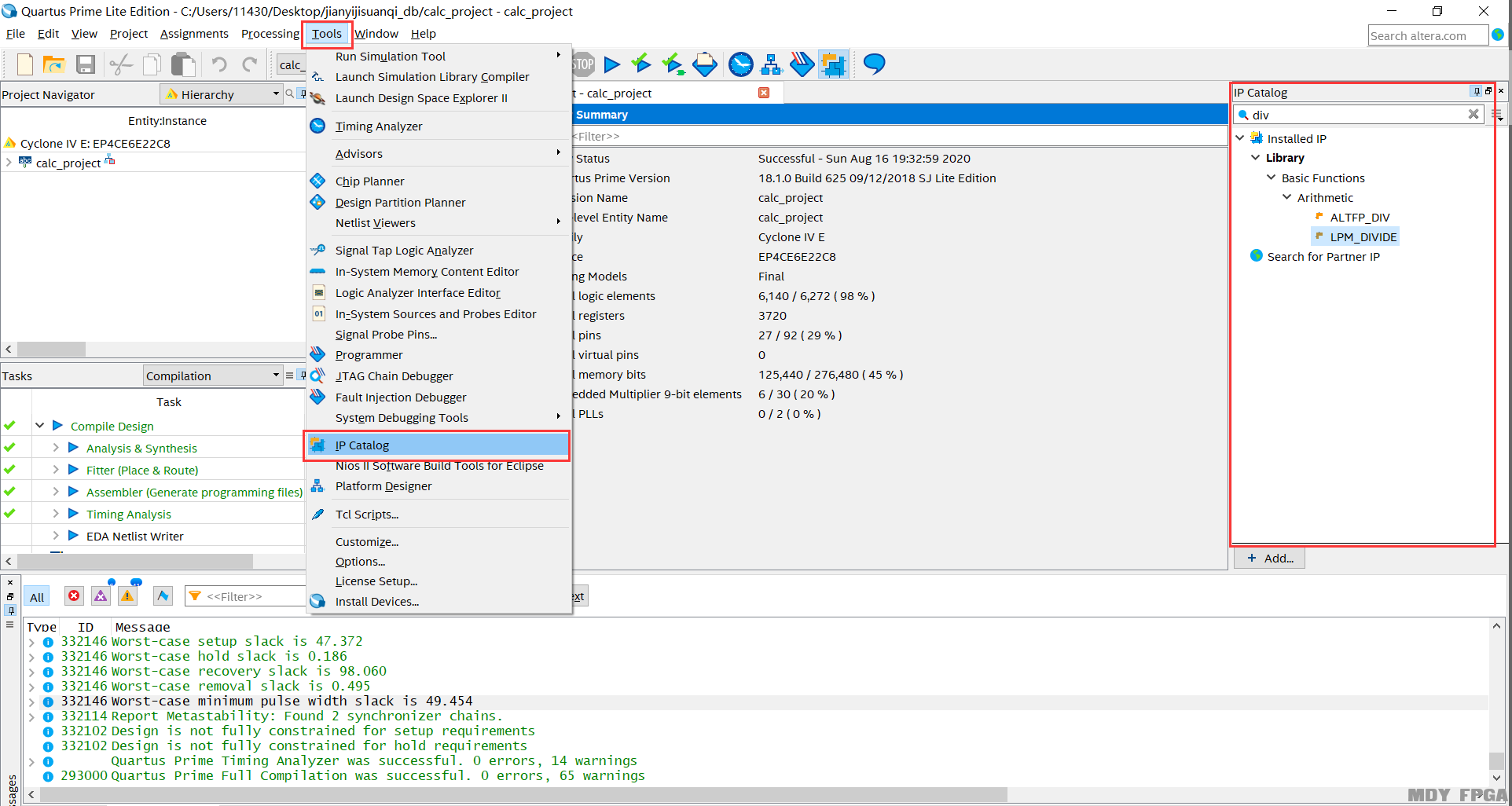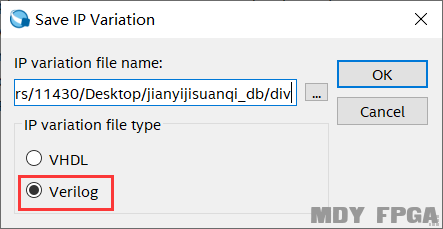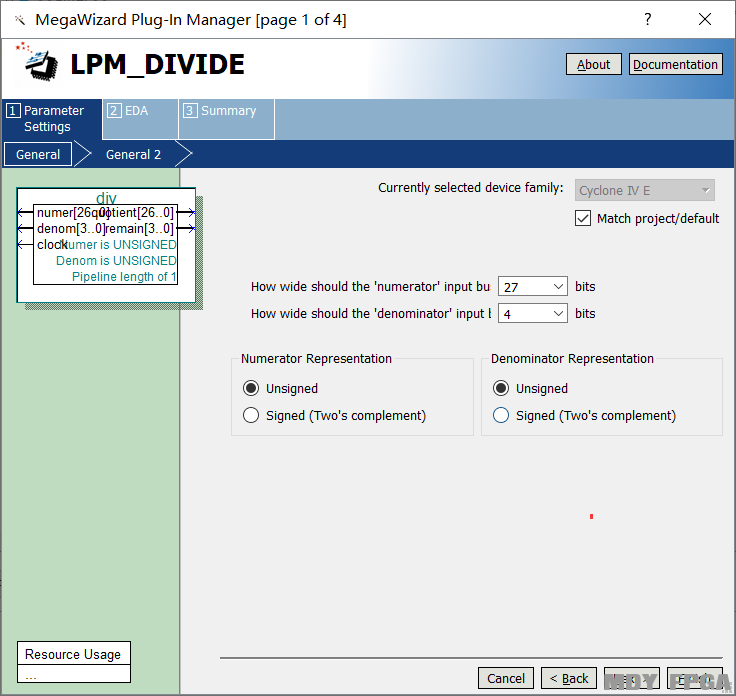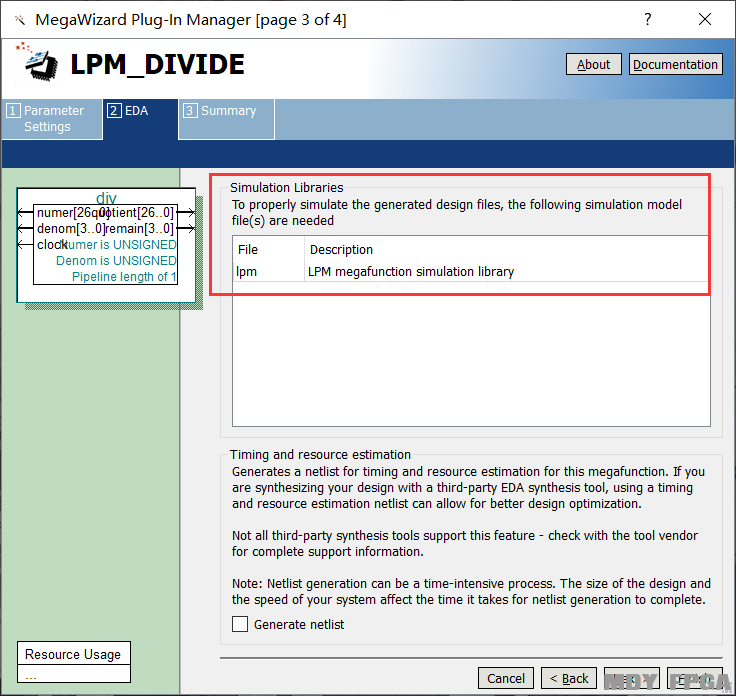1.8.3参考代码
1. always  @(posedge clk or negedge rst_n)begin
2.     if(rst_n==1'b0)begin
3.         result_neg_tmp <= 0;
4.     end
5.     else begin
6.         result_neg_tmp <= result_neg;
7.     end
8. end

9. always  @(*)begin
10.     if(state_c==OP_2)begin
11.         dis_sel = op_2;
12.     end
13.     else begin
14.         dis_sel = op_1;
15.     end
16. end

17. always  @(posedge clk or negedge rst_n)begin
18.     if(rst_n==1'b0)begin
19.         dis_sel_tmp <= 0;
20.     end
21.     else begin
22.         dis_sel_tmp <= dis_sel;
23.     end
24. end

25. div div_prj(
26.             .clock      (clk        )   ,
27.             .numer      (dis_tmp    )   ,
28.             .denom      (10         )   ,
29.             .quotient   (div_quo    )   ,
30.             .remain     (div_rem    )
31.             );

32. always  @(posedge clk or negedge rst_n)begin
33.     if(rst_n==1'b0)begin
35.     end
36.     else if(dis_sel!=dis_sel_tmp || result_neg!=result_neg_tmp)begin
38.     end
39.     else if(end_dis_cnt)begin
41.     end
42. end

43. always @(posedge clk or negedge rst_n) begin
44.     if (rst_n==0) begin
45.         dis_cnt <= 0;
46.     end
48.         if(end_dis_cnt)
49.             dis_cnt <= 0;
50.         else
51.             dis_cnt <= dis_cnt+1 ;
52.    end
53. end
55. assign end_dis_cnt = add_dis_cnt  && dis_cnt == 10-1 ;

56. assign  dis_tmp = add_dis_cnt && dis_cnt==1 ? dis_sel : div_quo;

57. always  @(posedge clk or negedge rst_n)begin
58.     if(rst_n==1'b0)begin
59.         display <= 4'b0;
60.     end
61.     else if(state_c==ERROR)begin
62.         display[4*(dis_cnt)-1 -:4] <= 4'b1111;
63.     end
64.     else if(end_dis_cnt && result_neg==1 && state_c!=OP_2)begin
65.         display[31:28] <= 4'b1010;
66.     end
67.     else begin
68.         display[4*(dis_cnt-1)-1 -:4] <= div_rem;
69.     end
70. end

71. always  @(posedge clk or negedge rst_n)begin
72.     if(rst_n==1'b0)begin
73.         display_vld <= 0;
74.     end
75.     else begin
76.         display_vld <= (dis_cnt==0 && (dis_sel==dis_sel_tmp)) ? 1'b1 : 1'b0;
77.     end
78. end

1.9 数码管显示模块设计1.9.1接口信号
 信号 接口方向 定义 clk 输入 系统时钟 rst_n 输入 低电平复位信号 Display 输入 显示数据输入信号 Display_vld 输入 显示数据有效指示信号 Seg_sel 输出 数码管位选信号 Segment 输出 数码管段选信号

1.9.2设计思路

1、  复位后，数码管默认显示运算数1；
2、  当result_err有效时，数码管显示8个F；
3、  当result_neg有效时，第8个数码管显示“—”；
4、  数码管显示display；

1.9.3参考代码
1. always @(posedge clk or negedge rst_n) begin
2.     if (rst_n==0) begin
3.         count_20us <= 0;
4.     end
6.         if(end_count_20us)
7.             count_20us <= 0;
8.         else
9.             count_20us <= count_20us+1 ;
10.    end
11. end
13. assign end_count_20us = add_count_20us  && count_20us == TIME_20US-1 ;

14. always @(posedge clk or negedge rst_n) begin
15.     if (rst_n==0) begin
16.         sel_cnt <= 0;
17.     end
19.         if(end_sel_cnt)
20.             sel_cnt <= 0;
21.         else
22.             sel_cnt <= sel_cnt+1 ;
23.    end
24. end
26. assign end_sel_cnt = add_sel_cnt  && sel_cnt == SEG_NUM-1 ;

27. always  @(posedge clk or negedge rst_n)begin
28.     if(rst_n==1'b0)begin
29.         seg_sel <= {SEG_NUM{1'b1}};
30.     end
31.     else begin
32.         seg_sel <= ~(1'b1 << sel_cnt);
33.     end
34. end

35. always  @(posedge clk or negedge rst_n)begin
36.     if(rst_n==1'b0)begin
37.         display_ff0 <= 0;
38.     end
39.     else begin
40.         for(ii=0;ii<SEG_NUM;ii=ii+1)begin
41.             if(display_vld==1)begin
42.                 display_ff0[(ii+1)*4-1 -:4] <= display[(ii+1)*4-1 -:4];
43.             end
44.             else begin
45.                 display_ff0[(ii+1)*4-1 -:4] <= display_ff0[(ii+1)*4-1 -:4];
46.             end
47.         end
48.     end
49. end

50. always  @(*)begin
51.     seg_tmp = display_ff0[(sel_cnt+1)*4-1 -:4];
52. end

53. always  @(posedge clk or negedge rst_n)begin
54.     if(rst_n==1'b0)begin
55.         segment <= NUM_0;
56.     end
57.     else begin
58.         case(seg_tmp)
59.             0 :segment <=NUM_0  ;
60.             1 :segment <=NUM_1  ;
61.             2 :segment <=NUM_2  ;
62.             3 :segment <=NUM_3  ;
63.             4 :segment <=NUM_4  ;
64.             5 :segment <=NUM_5  ;
65.             6 :segment <=NUM_6  ;
66.             7 :segment <=NUM_7  ;
67.             8 :segment <=NUM_8  ;
68.             9 :segment <=NUM_9  ;
69.             10:segment <=NUM_10 ;
70.            default:segment <= NUM_ERR;
71.        endcase
72.     end
73. end

1.10 蜂鸣器模块设计1.10.1接口信号
 信号 接口方向 定义 clk 输入 系统时钟 rst_n 输入 低电平复位信号 Op_1_err 输入 运算数1溢出信号，高电平有效 Op_2_err 输入 运算数2溢出信号，高电平有效 Result_err 输入 运算结果错误信号，高电平有效 Beep 输出 蜂鸣输出信号，高电平有效

1.10.2设计思路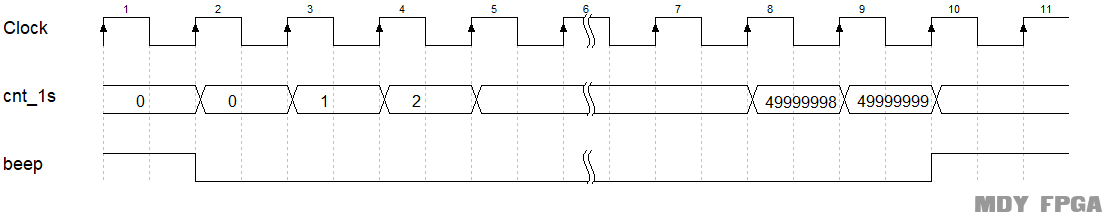1.10.3参考代码
1. always @(posedge clk or negedge rst_n) begin
2.     if (rst_n==0) begin
3.         cnt_1s <= 0;
4.     end
6.         if(end_cnt_1s)
7.             cnt_1s <= 0;
8.         else
9.             cnt_1s <= cnt_1s+1 ;
10.    end
11. end
13. assign end_cnt_1s = add_cnt_1s  && cnt_1s == CNT_1S-1 ;

14. always  @(posedge clk or negedge rst_n)begin
15.     if(rst_n==1'b0)begin
16.         beep <= 1'b1;
17.     end
18.     else if(op_1_err || op_2_err || result_err)begin
19.         beep <= 1'b0;
20.     end
21.     else if(end_cnt_1s)begin
22.         beep <= 1'b1;
23.     end
24. end

1.11 效果和总结

1.11.1db603开发板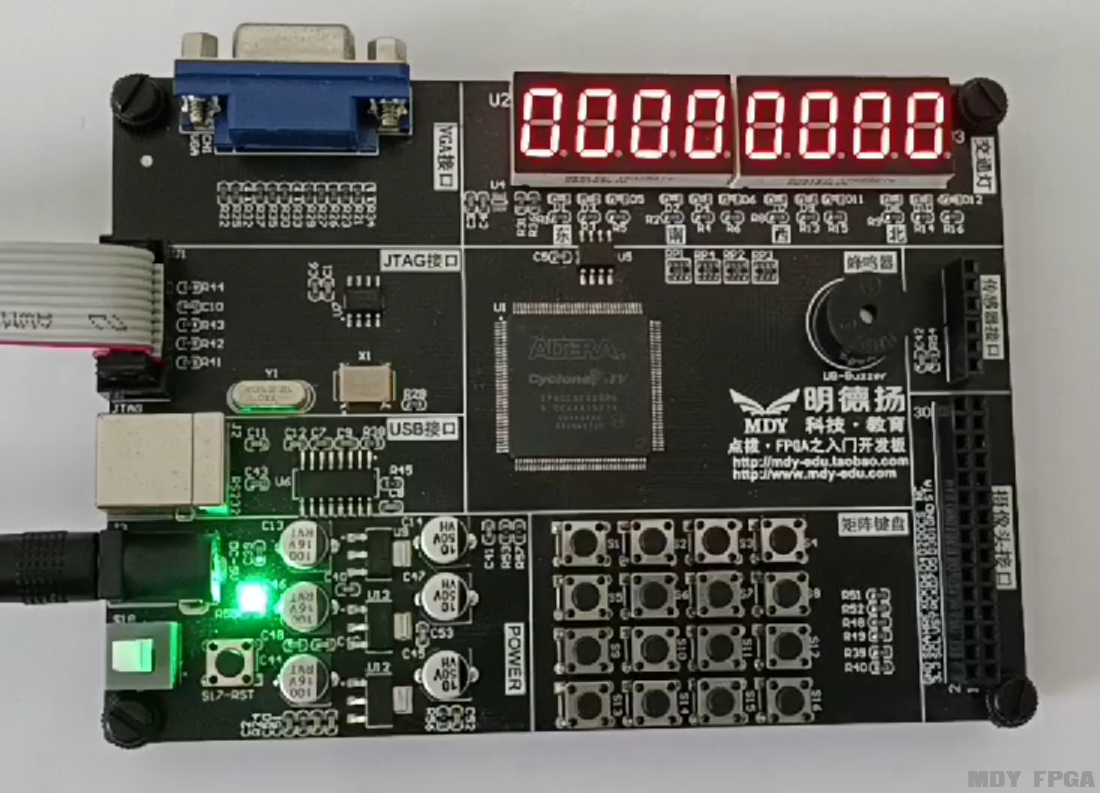1.11.2ms980试验箱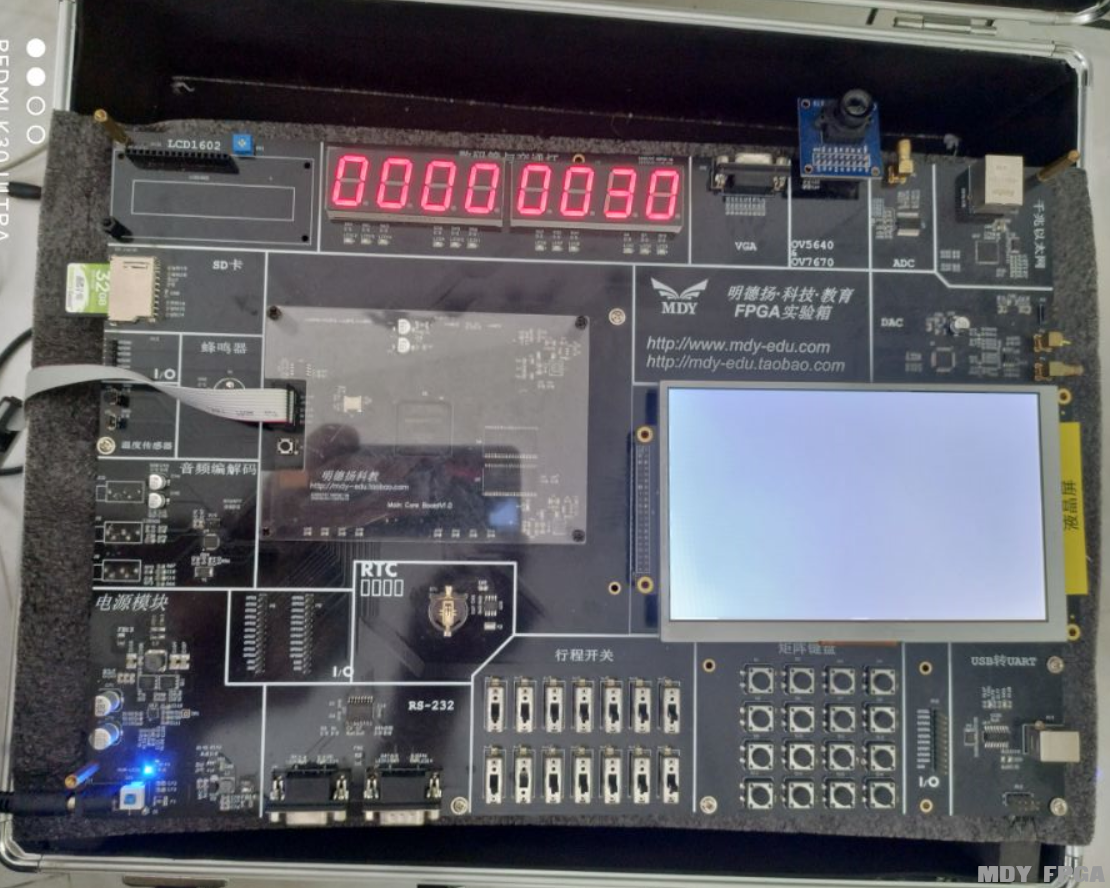1.12 公司简介

MP801

# 报名FPGA培训

•
•
•
•
• FPGA教育领域第一品牌
• 咨询热线：020-39002701
• 技术交流Q群：544453837